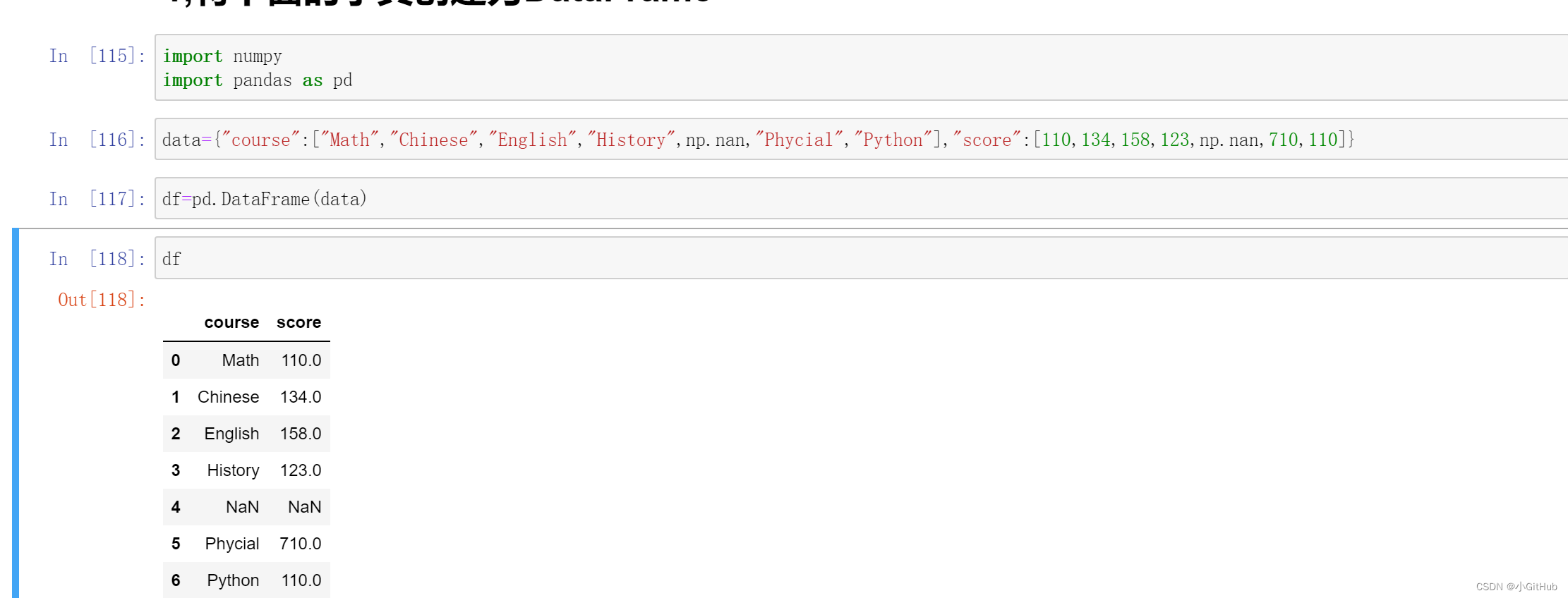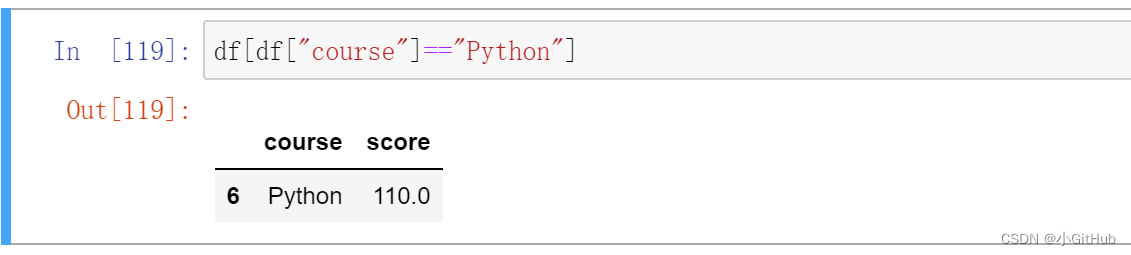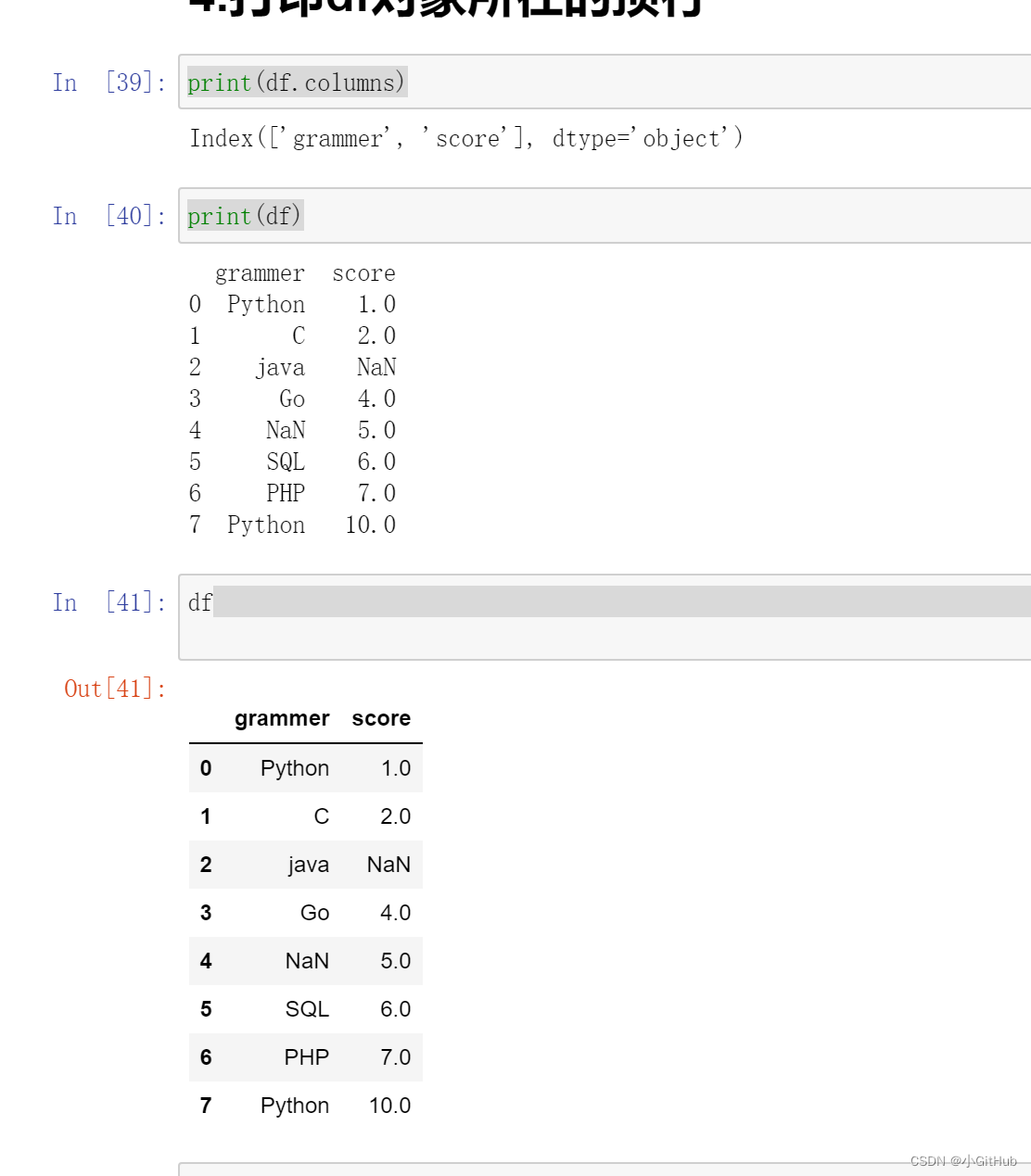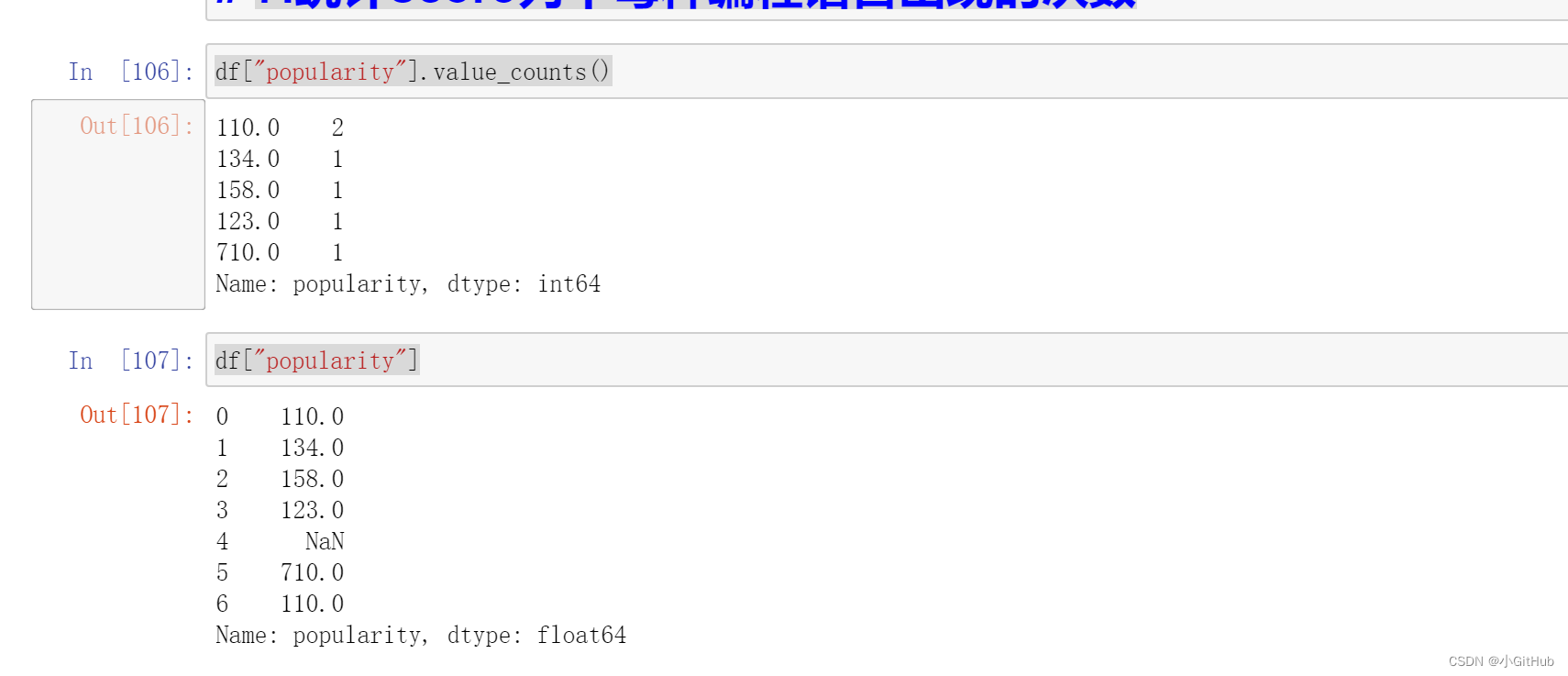# Python 学习基础pandas刷题（一）

### 1,将下面的字典创建为DataFrame

``````import numpy

import pandas as pd

data={"course":["Math","Chinese","English","History",np.nan,"Phycial","Python"],"score":[110,134,158,123,np.nan,710,110]}

df=pd.DataFrame(data)

df

course	score
0	Math	110.0
1	Chinese	134.0
2	English	158.0
3	History	123.0
4	NaN	NaN
5	Phycial	710.0
6	Python	110.0``````

###import
import()是python的一个内置函数，用于动态加载类和函数。
numpy
numpy(Numerical Python) 是 Python 语言的一个扩展程序库，支持大量的维度数组与矩阵运算，此外也针对数组运算提供大量的数学函数库。

pandas
pandas是python的一个数据分析包，提供了大量能使我们快速便捷地处理数据的函数和方法。
as

注释2:

DataFrame 一个表格型的数据结构，既有行标签（index），又有列标签（columns），它也被称异构数据表，所谓异构，指的是表格中每列的数据类型可以不同，比如可以是字符串、整型或者浮点型等。

### 2.提取含有字符串“course列为Python”的行

``````df[df["course"]=="Python"]

course	score
6	Python	10.0``````

###df["course"]可以访问到course所在列在所有元素，而df访问到整个表格。

### 3.提取分值为110所在的行¶

``````df[df["score"]==110]

course	score
0	Math	110.0
6	Python	110.0``````

### 4.打印df对象所在的列标签

``````print(df.columns)

Index(['grammer', 'score'], dtype='object')

print(df)

grammer  score
0  Python    1.0
1       C    2.0
2    java    NaN
3      Go    4.0
4     NaN    5.0
5     SQL    6.0
6     PHP    7.0
7  Python   10.0``````

###df.columns访问到所在的列标签

### 5.修改第二列名成为“popularity”

``````df.rename(columns={"score":"popularity"},inplace=True)

df

course	popularity
0	Math	110.0
1	Chinese	134.0
2	English	158.0
3	History	123.0
4	NaN	NaN
5	Phycial	710.0
6	Python	110.0``````

### 6.统计course列中每种编程语言出现的次数

``````df["course"].value_counts()

Math       1
Chinese    1
English    1
History    1
Phycial    1
Python     1
Name: course, dtype: int64
``````

### 7.统计popularity列中每种编程语言出现的次数

``````df["popularity"].value_counts()

110.0    2
134.0    1
158.0    1
123.0    1
710.0    1
Name: popularity, dtype: int64

df["popularity"]

0    110.0
1    134.0
2    158.0
3    123.0
4      NaN
5    710.0
6    110.0
Name: popularity, dtype: float64``````df.value_counts()可以计算出每行出现的次数，同理df["popularity"].value_counts()可以计算出popularity列中每行出现的次数。

rename表示重新命名，df.rename(columns={"A":"B"})将A标签修改成B标签。